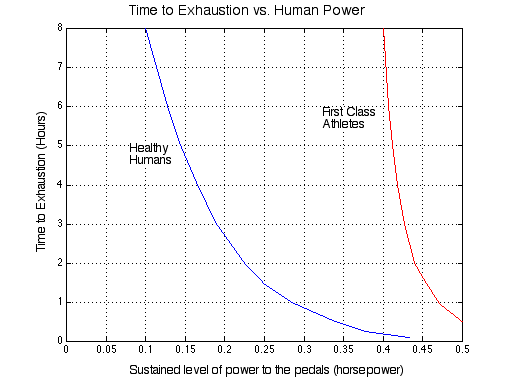### The Steady State Power Equation of Cycling

The word statement of the basic power equation is:Recall that the kinetic energy of a mass m moving with velocity V is given by:The inertial power is given by the time derivative of the kinetic energy, thus:Thus the complete power equation is given by:``

Note that the above equation is a nonlinear differential equation in velocity V and can only be solved by numerical techniques for the nonsteady conditions of acceleration or deceleration.
Under steady state (constant velocity) the power equation can be written as follows:### The Power Calculator

 Your weight (in kg) Bicycle weight (in kg) Bicycle velocity (in km/h) Headwind velocity (in km/h) Air density (in kg/m3) Effective frontal area (in m2) Coefficient of rolling resistance Road Slope (in %) Gravitation (in m/s2) Required Total Power (in Watt) - Power to fight air resistance - Power to fight gravity

### The Human Engine

Consider now the human engine. In 1983 Douglas Malewicki gave a landmark paper at the International Human Powered Vehicle Association Scientific Symposium, in which he presented a graph showing the maximum duration of human effort for various steady power levels. This graph has been reproduced below for convenience. Notice from the graph that an average "healthy human" can produce a steady 0.1 horsepower (75 Watt) for a full eight hour period, while a "first class athlete" can produce 0.4 horsepower (300 Watt) for a similar period. Note that each data point on the curves represents an exhausted human. No more power is available without some rest and recovery. Thus at 0.4 hp the "healthy human" becomes exhausted within 10 minutes! Try to decide where you fit between these curves.Back to Index